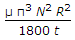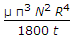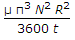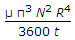# Mechanical Engineering - Hydraulics and Fluid Mechanics

### Exercise :: Hydraulics and Fluid Mechanics - Section 4

11.

A flow whose streamline is represented by a curve, is called

 A. one-dimensional flow B. two-dimensional flow C. three-dimensional flow D. four-dimensional flow

Answer: Option B

Explanation:

No answer description available for this question. Let us discuss.

12.

When the Mach number is less than unity, the flow is called

 A. sub-sonic flow B. sonic flow C. super-sonic flow D. hyper-sonic flow

Answer: Option A

Explanation:

No answer description available for this question. Let us discuss.

13.

When the end contractions of the weir are suppressed, then number of end contractions (n) are taken as zero

 A. Agree B. Disagree

Answer: Option A

Explanation:

No answer description available for this question. Let us discuss.

14.

A flow is called hyper-sonic, if the Mach number is

 A. less than unity B. unity C. between 1 and 6 D. none of these

Answer: Option D

Explanation:

No answer description available for this question. Let us discuss.

15.

The power absorbed (in watts) in overcoming the viscous resistance of a footstep bearing is

 A.B.C.D.Answer: Option B

Explanation:

No answer description available for this question. Let us discuss.

#### Current Affairs 2021

Interview Questions and Answers